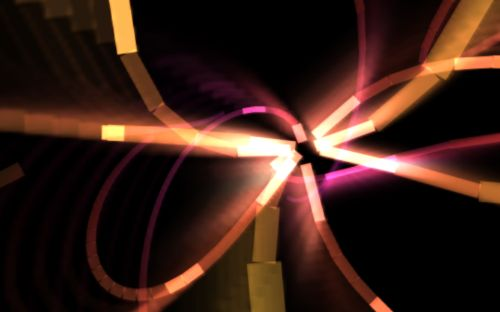# 1k Javascript Demo ContestPulsing 3D wires with volumetric beams

JS1K is a Javascript demo contest where the max size of the demo must not exceed 1k (or 1024 characters). Some of the demos can even fit in a tweet (140 characters) like this one: Binary clock. Here is the code source of this tweetable demo:

```setInterval("
x=document.body.children.getContext('2d');
x.clearRect(0,0,o=99,9);
d=+new Date()*2;
while(d=d/2)x.fillRect(o-=3,0,d&1*2,9)
",9)
```

Here is the code of the Pulsing 3D wires demo (1024 char):

```f=Math;
e=document.body.children[\$=0];
G="globalCompositeOperation";
Q=.43;
P=.05;
with(e)
{
with(style)width=(w=innerWidth-9)+"px",height=(h=innerHeight-25)+"px";
W=(width=w/=2)/2;
H=(height=h/=2)/2;
g=getContext("2d");
t=w/h
}
with(g)
{
scale(W/t,H);
translate(t,1);
setInterval(
function()
{
with(E=e.cloneNode(0))width=height=H,c=getContext("2d");
c.fillRect(0,0,h,h);
g[G]=c[G]="lighter";
C=f.cos;
S=f.sin;
L=f.atan2;
q=C(\$);
r=S(q-\$*.7)+Q;
u=C(r-\$*Q)+Q;
a=L(q,-u*2);
b=L(r,u*u+q*q);
n=C(a);
o=S(a);
N=C(b);
O=S(b);
\$+=P;
clearRect(-t,-1,2*t,2);
for(i=14;i>4;--i)
{
v=0;
for(j=25;j;)
{
M=f.log(j+.2)*Q;
j--;
_=\$-j*.07-i*4;
A=C(_+S(_*.8))*2+_*P;
B=S(_*.7-C(_*Q))*3;
x=C(A)*C(B)*M-q;
y=S(A)*C(B)*M-r;
z=S(B)*M-u;
k=x*n+z*o;
_=z*n-x*o;
l=y*N+_*O;
z=_*N-y*O;
lineTo(k/=z,l/=z);
lineWidth=P/z;
strokeStyle="hsl("+60*S(\$-z)+",60%,"+~~(40-j)*(Q+!j+(.1>(\$-j*P)%1))+"%)";
if(z>.1)v++&&stroke();
else{v=0}beginPath();
moveTo(k,l)
}
}
A="drawImage";
N=H/2;
c.globalAlpha=Q;
c[A](e,0,0,H,H);
X=k*N+N;
Y=l*N+N;
K=1.1;
c.translate(X,Y);
while(i--)c.scale(K,K),c[A](E,-X,-Y,H,H);
g[A](E,-t,-1,2*t,2)
}
,33)
}
```

## One thought on “1k Javascript Demo Contest”

1.auld

I think this was a fantastic sizecoding contest, hugely successful with some great entries. And it wasnt demoscene! Some of the entries were stunning. My daughter played the winning entry for >1 hour. My only criticism is that all the winners were games and I believe this shows unintended bias. Judges should have picked at least one non-game out of those amazing entries.